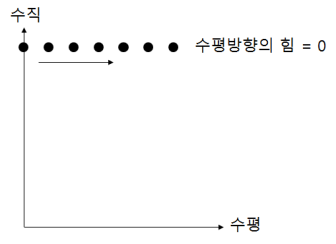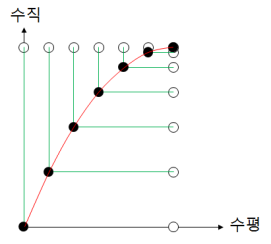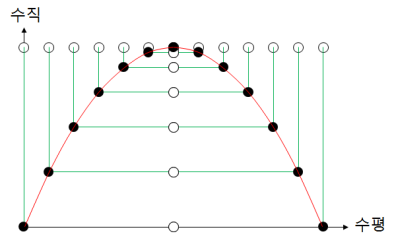# Clay Shooting

Projectile motion

Let’s consider the movement of throwing an object horizontally.
Assuming no air resistance, there is no horizontal force, so no change in horizontal speed.
In other words, it’s constant velocity linear motion in the horizontal direction.This time, let’s consider a free fall motion that drops vertically.
In the vertical direction, there is a change in velocity due to the Earth’s gravity. In other words, ‘uniformly accelerated motion’ will be occur.Assuming that an object is dropped from an airplane, it will be a constant velocity linear motion in the horizontal direction and will be a free fall motion in the vertical direction.
The final motion of an object can be obtained by combining horizontal motion and vertical motion.What happens if you throw an object at an angle?When you throw an object at an angle, the force exerted on the object is only Earth’s gravity. As a result, it moves along a parabolic orbit.